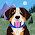## Karl's Rate Problem

Unit Rate to Rate

My Problem

If there's 12 pigs per 10 sheep, how many pigs if there's only one sheep?

Group Problem

If there is 2 cookies per milk, how many cookies do they need in 10 milk?

Rate to Unit Rate

My Problem

If there's 20 pizzas per 1 people, how many pizzas per 13 people?

Group Problem

There are 10 chairs per 5 tables, how many chairs are there per 2 table?

7:14 PM

#### 4 comments :

1.ok Karl here is my problem for you
if there are 15 chickens per 20 pigs, how many chickens if there is only three pigs

1.ok Thank You justin

2.Here's the answer for your last question:
~If there's 2 tables there would be 4 chairs. So its 4 chairs per 2 tables.

Here's a question:
~There are 12 chairs per 6 tables, how many chairs are there per 3 table?

1.